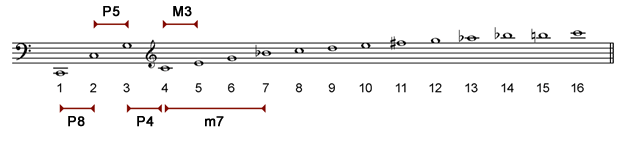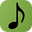When we hear a sound produced by a musical instrument we are actually listening to a multiplicity of sounds that form the harmonic series. In the seventeenth century French Joseph Sauveur (1653-1716) and the Englishman Thomas Pigot (1657-1686) noted that the strings vibrate in sections, a phenomenon that explains why one string produces this multiplicity of sounds.

The video below shows the spectrum analysis of a C two octaves below middle C (C2):

Notes:

• We have written the musical notes corresponding to the first 16 harmonics of the series. It is obvious that many harmonics follow these first harmonics.
• The first harmonic or fundamental is not necessarily the strongest harmonic.
• The balance between the harmonics varies constantly. This coupled with the immense amount of harmonics explains the difficulty we encounter in synthesizing sounds.

Importance of the harmonic series

The harmonic series defines many of our intervals. Listed below are the octave, fifth, fourth, major third and minor seventh:We can calculate mathematical ratio (or size) by dividing the frequencies of notes. Here we use the frequency of some harmonics to calculate the size of intervals:Interval Ratio From the harmonics
Octave 130 / 65 = 2 1 and 2
Fifth 195 / 130 = 1.5 2 and 3
Fourth 260 / 195 = 1.33 3 and 4
Major third 325 / 260 = 1.25 4 and 5
Minor seventh 455 / 260 = 1.75 4 and 7

Interestingly we can calculate the values using harmonic numbers:

Interval Ratio From the harmonics
Octave 2 / 1 = 2 1 and 2
Fifth 3 / 2 = 1.5 2 and 3
Fourth 4 / 3 = 1.33 3 and 4
Major third 5 / 4 = 1.25 4 and 5
Minor seventh 7 / 4 = 1.75 4 and 7

Calculating frequencies

The mathematical ratios can be used to calculate the frequency of notes. From an A 440 we calculate the frequency of C#, E and G:

 A C# (major third) E (perfect fifth) G (minor seventh) 440 440 x 1.25 = 550 440 x 1.5 = 660 440 x 1.75 = 770

If we divide by the mathematical ratio we obtain descending intervals. Here we calculate the frequency of an F, a major third below A: